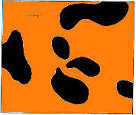# The Tiger's Stripes

A technical blog on math, computer science, and game theory.

Author: Bo Waggoner RSS feed# Eliciting Finite Properties

Posted: 2017-04-22.

In this post we'll look at eliciting "finite properties" of distributions in other words, multiple-choice questions about some unknown or future event. This is a continuation of the series on elicitation.

## Elicitation Recap

There is a random variable $Y$ taking values in the set $\mathcal{Y}$. An "expert" makes some report $r \in \mathcal{R}$, interpreted as some sort of prediction about $Y$. In this post, $\mathcal{R}$ is a finite set, interpreted as asking the agent a multiple-choice question about $Y$.

What does it mean to answer such a question truthfully? This is formalized by a property, a function $\Gamma: \Delta_{\mathcal{Y}} \to 2^{\mathcal{R}}$, where $\Gamma(p)$ is the set of allowable or truthful reports for belief $p$. We say $\Gamma$ is elicitable if there exists a scoring rule $S: \mathcal{R} \times \mathcal{Y} \to \mathbb{R}$ such that $$\Gamma(p) = \arg\max_{r\in\mathcal{R}} \mathbb{E}_p S(r,y) .$$

The level set of report $r$ is $\{p: r \in \Gamma(p)\}$. In the previous post, we proved (well, the reader was asked to prove) that if $\Gamma$ is elicitable, then each level set is convex.

## General Characterization Recap

Rather than just pointing to the previous post, let's give a somewhat different form of the general characterization.

Theorem. $\Gamma$ is elicitable if and only there exists a convex function $G: \Delta_{\mathcal{Y}} \to \mathbb{R}$ and a set of hyperplanes $H = \{h_r : r \in \mathcal{R}\}$ such that:

1. $G(p)$ is the expected score for reporting truthfully given belief $p$.
2. $G$ is the pointwise maximum of $H$.
3. $G$ equals $h_r$ at exactly the level set of $r$.
4. The scoring rule $S$ eliciting $\Gamma$ has $S(r,y)$ equal to $h_r$ at $\delta_y$; that is, the height of the hyperplane $h_r$ above the point $\delta_y$, which is the distribution with mass one on $y$.

## The Finite Properties Case

In the case of finite properties, $\mathcal{R}$ is restricted to be a finite set, which makes $G$ the pointwise maximum of a finite set of hyperplanes. This imposes a very special structure on the kinds of level sets, and therefore the kinds of properties, that are elicitable. Let's see some examples first, starting with one from the previous post: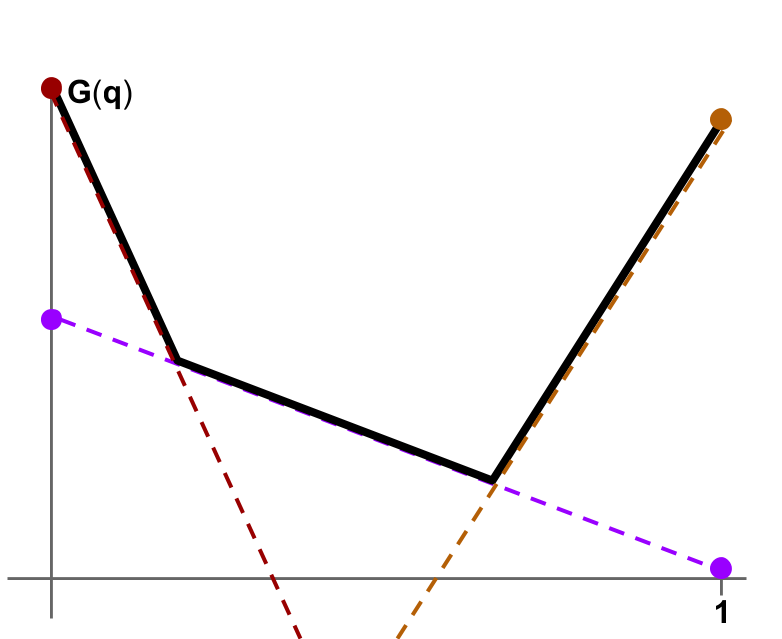The event $\mathcal{Y}$ has two outcomes, with the horizontal axis plotting the probability of the first outcome. $\Gamma$ has three reports: low'', medium'', and high'' probability, each corresponding to some hyperplane.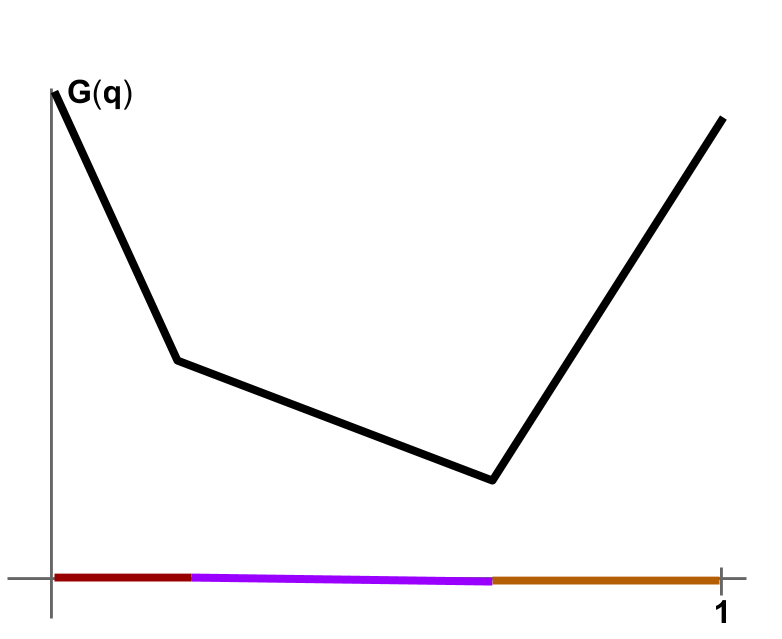The three level sets, i.e. the sets of distributions corresponding to each report. Each is the set of distributions above which the hyperplane for that report touches $G$.

The next set of examples are finite properties of distributions on three outcomes, so $G$ will be a convex function from $\Delta_3$ to $\mathbb{R}$. We can plot $\Delta_3$ (the set of probability distributions on three outcomes) as an equilateral triangle in the plane. Code here.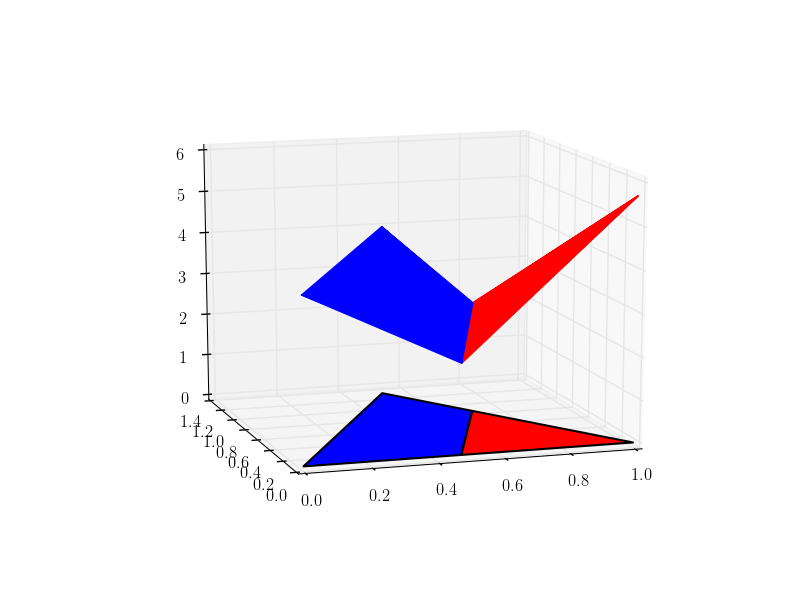A convex function $G$ as the maximum over a set of different hyperplanes, each corresponding to a different report (each with a different color). The corresponding level sets for each report -- the set of distributions for which that report is truthful -- give a partition of the simplex, with matching colors.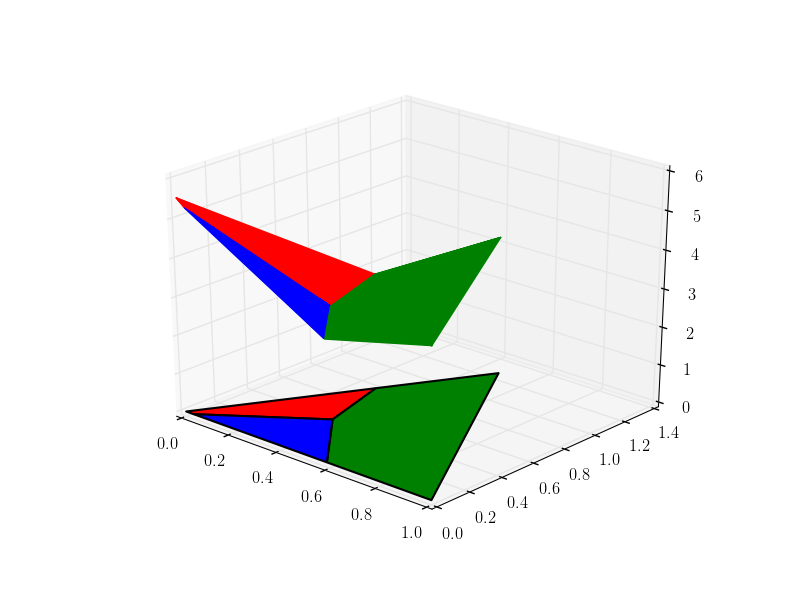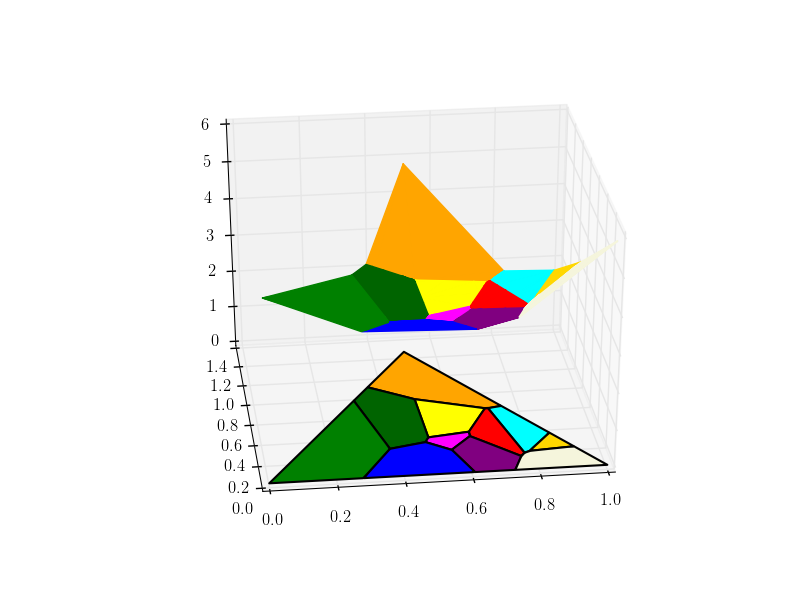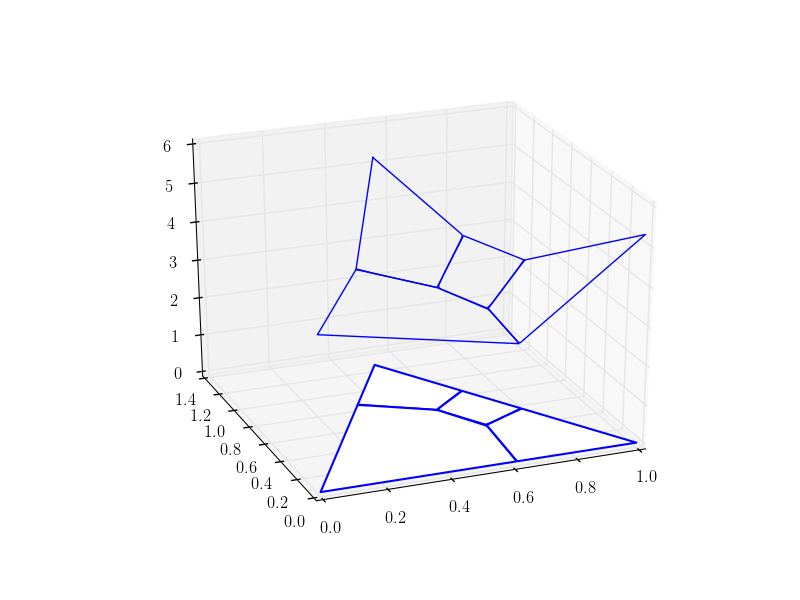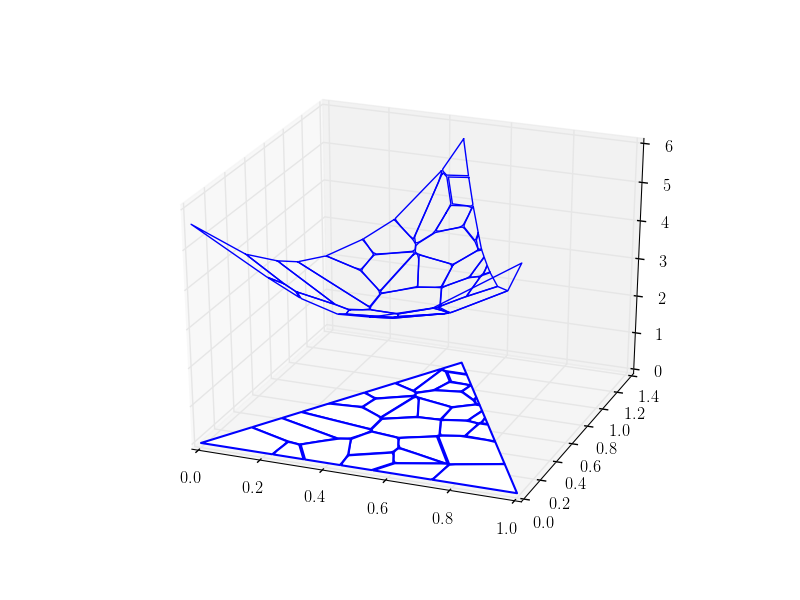Why someone would be interested in eliciting these particular properties, I do not know.

## Finite Properties Characterization

The finite-properties variant of the problem was introduced by Lambert and Shoham (2009) (see also Lambert's updated 2013 paper), who proved a nice characterization. First we need a definition.

Definition. A power diagram of a convex set (e.g. the simplex) is a finite set of regions $\{A_r : r \in \mathcal{R}\}$ satisfying the following conditions. Let $A(p) = \{r: p \in A_r\}$; then $A(p)$ is nonempty and, for some set of points $\{d_r : r \in \mathcal{R}\}$ and constants $\{c_r : r \in \mathcal{R}\}$, $$A(p) = \arg\min_{r \in \mathcal{R}} ~ \|p - d_r\|_2^2 + c_r .$$

One note: sometimes the definition of power diagram requires all $c_r \geq 0$, but the above definition is equivalent: we can shift every $c_r$ by the same amount without changing the regions, until all $c_r$ are positive.

Here's a useful lemma that is probably known or could be found by asking the right experts.

Lemma. The regions $\{A_r\}$ can form a power diagram for points $\{d_r\}$ if and only if there exists a convex function $G$, the pointwise maximum over a finite set of affine functions, such that $p \in A_r$ if and only if $d_r$ is a subgradient of $G$ at $p$.

Proof. Suppose $\{A_r\}$ do form a power diagram for $\{d_r\}$ and $\{c_r\}$. We have, making transformations that do not affect the argmin: \begin{align} A(p) &= \arg\min_r \|p\|_2^2 - 2 p \cdot d_r + \|d_r\|_2^2 + c_r \\ &= \arg\min_r \left(\|d_r\|_2^2 + c_r\right) - 2 p \cdot d_r \\ &= \arg\min_r c_r' - p \cdot d_r \\ &= \arg\max_r p \cdot d_r - c_r' \end{align} where $c_r' = 0.5 \left(\|d_r\|_2^2 + c_r\right)$.

In particular, if we let $G(p) = \max_r p \cdot d_r - c_r'$, then $G(p)$ is a pointwise maximum over affine functions, hence is convex, and by what we know of convex duality, the argmax is achieved precisely when $d_r$ is a subgradient of $G$ at $p$.

Now suppose $G$ is given as a pointwise maximum over the affine functions $\{p \mapsto p \cdot d_r - c_r'\}$ for some vectors $\{d_r\}$ and scalars $\{c_r'\}$. Then by the same calculations above, the level sets of the subgradient map -- i.e. each $A_r$ contains $p$ if and only if $d_r \in \partial G(p)$ -- form a power diagram with points $\{d_r\}$ and some $c_r$ which can be computed from $c_r'$.

This gives a slightly but not essentially different proof of Lambert and Shoham's result.

Theorem. A finite property $\Gamma$ is elicitable if and only if its level sets form a power diagram.

Proof. The same characterization applies to both.

Power diagrams are related to the better-known Voronoi diagrams, where we are given a finite set of reference points $\{d_r\}$ and partition space into cells via the closest reference point. In particular, a power diagram on an $n-1$ dimensional plane or convex subset thereof, such as $\Delta_n$ (the simplex on $n$ outcomes), can always be formed by taking a Voronoi diagram in $n$ dimensional space and taking its intersection with that plane. So we can phrase the characterization in this way as well. (For me, the picture is worth, well, let's say at least a dozen characterizations.)

(2009) Nicolas Lambert and Yoav Shoham. Eliciting truthful answers to multiple-choice questions. EC '09.